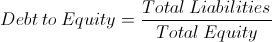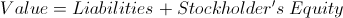# Debt to Equity Ratio (D/E)The formula for the debt to equity ratio is total liabilities divided by total equity. The debt to equity ratio is a financial leverage ratio. Financial leverage ratios are used to measure a company's ability to handle its long term and short term obligations.

Both debt and equity will be found on a company's balance sheet. Debt may show as total liabilities and equity may show as total stockholder's equity.

## Underlying Concept of Debt to Equity Ratio

Understanding the debt to equity ratio first requires understanding the capital structure of a company. A company's value, or at least as it is denoted based on assets, is a company's liabilities plus stockholder's equity, or owner's equity.Considering that a company's assets or value is comprised of liabilities plus equity, the debt to equity ratio contrasts these two variables to show a company's leverage position. As a company increases their leverage or debt, they are considered a higher risk. This higher risk and debt to equity ratio is discussed in the following section.

## Use of the Debt to Equity Ratio

The debt to equity ratio is a particularly important financial leverage ratio, in that it is used to calculate levered beta, which is sometimes referred to as equity beta. As the number of formulas, as well as variables, required to complete a specific formula increase, the more variance in results occur due to errors. This is especially true for the debt to equity ratio as a company's capital structure decisions rely upon an accurate understanding of the current state of its financial affairs.

For example, as the debt of a company increases, the debt to equity ratio increases as well. In turn, the levered beta of a company increases due to the debt to equity ratio increasing. This is particularly important issue when companies make decisions of whether to issue new debt or issue new equity for financing. Incurring new debt will increase the risk or levered beta of a company, ceteris paribus.

New to Finance?